• 粒子滤波在GPS 动态滤波中的应用，周建国，，本文给出了粒子滤波的GPS动态滤波的算法。在GPS导航系统中存在的噪声对GPS导航精度存在比较大的影响。传统的卡尔曼滤波算法对GPS动态
•研究论文
• 论文题目：Dynamic Filter Networks ... summary 在传统的卷积层中，经过训练后的学习滤波器保持不变。相反本篇论文引入了一种动态过滤器网络，根据输入动态生成，但是却...动态滤波网络由过滤生成网络和动态过滤层组...
论文题目：Dynamic Filter Networks

论文链接：https://arxiv.org/pdf/1605.09673.pdf

summary

在传统的卷积层中，经过训练后的学习滤波器保持不变。相反本篇论文引入了一种动态过滤器网络，根据输入动态生成，但是却没有过多地增加模型参数的数量。

动态滤波网络组成

总体框架如下图所示：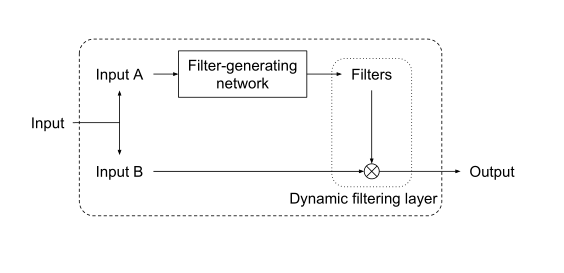动态滤波网络由过滤生成网络和动态过滤层组成。过滤生成器网络根据输入动态生成过滤器，而动态过滤层将生成的过滤器应用于另外一个输入。两部分都是可微的。

过滤生成网络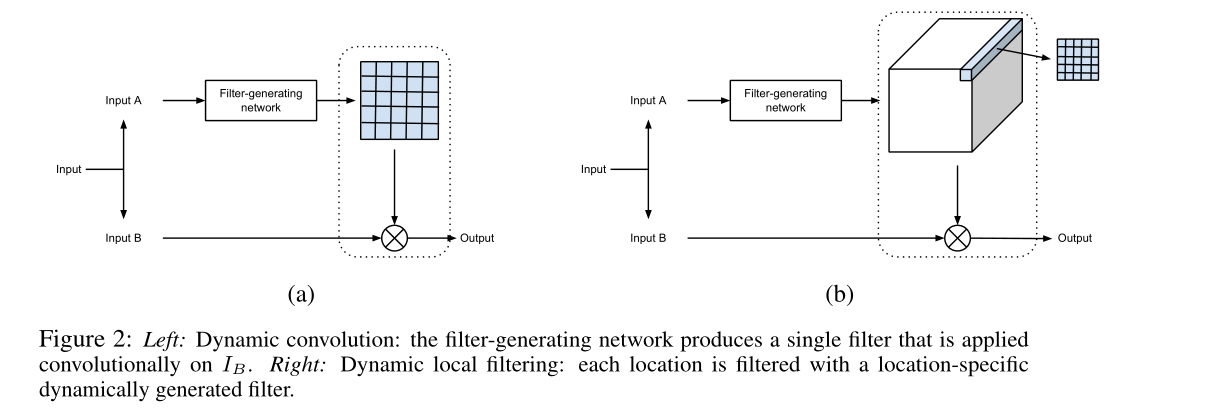过滤生成网络输入是：, h为高，w为宽，Ca为输入A通道的数目，输出为，,s为滤波器的尺寸，n为滤波器的数目，动态卷积d=1，Cb为输入B通道的数目，hxW为局部滤波。将过滤器应用于输入，输出。

过滤生成网络可以用任何可微分的架构来实现，比如多层感知器或卷积网络。当使用图像作为过滤器生成网络的输入时，卷积网络尤其适用。（使用编码器-解码器结构可以增加生成滤波器的卷积网络的接受域。我们还可以对过滤器生成网络的输出应用平滑惩罚，以便鼓励相邻的过滤器应用相同的转换。）

动态过滤层
动态卷积层：动态过滤层动态参数θ由过滤生成网络生成。

动态局部滤波层：在这个层中，过滤操作不再是平移不变量，不同的滤波器被应用到输入的不同位置上。对于输入的每个不同位置，一个特定的局部滤波器Fθ(i,j)被应用到以（i，j）为中心的区域。G(i, j) = Fθ(i,j)(IB(i, j))。动态局部滤波层不仅可以像动态卷积层那样执行单个的变换，还可以像局部变形那样执行特定位置的变换。与传统的局部连接层相比，动态局部滤波层的另一个优点是我们不需要太多的模型参数。所学习的模型更小，这是嵌入式系统应用所需要的。

Experiment实验

①学习可操纵滤波器

过滤生成网络的任务是将一个角度转换成一个过滤器，然后将其应用于输入图像以生成最终的输出。我们将滤波器生成网络实现为几个完全连接的层，最后一层包含81个神经元，对应于一个9x9卷积滤波器的元素。下图显示了一个经过训练的网络示例。它确实学习了期望的过滤器，并对图像应用了正确的转换。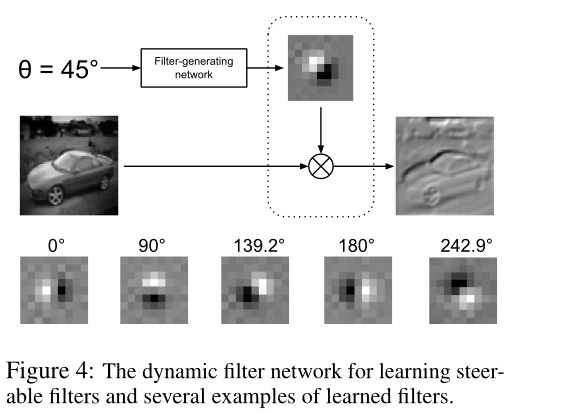②视频预测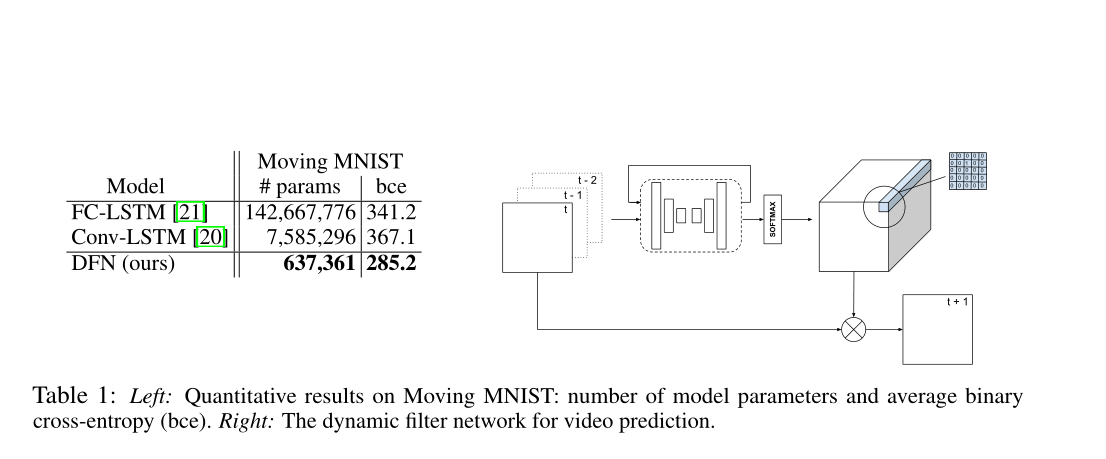论文使用卷积编译码器作为过滤生成网络，其中编码器由几个跨卷积层组成，而解码器由几个反池化层和卷积层组成。卷积编码器-解码器能够利用帧内的空间相关性，生成与帧大小相同的特征映射。每个生成的过滤器都应用了一个softmax层，这样每个过滤器都被鼓励只有几个非零元素。为了生成下一帧的预测，将生成的滤波器应用于前一帧，根据第3节中介绍的动态局部滤波机制对前一帧进行变换。使用softmax层可以帮助动态过滤层生成更清晰的图像，因为输出图像中的每个像素都来自前一帧中的几个像素。

③moving Mnist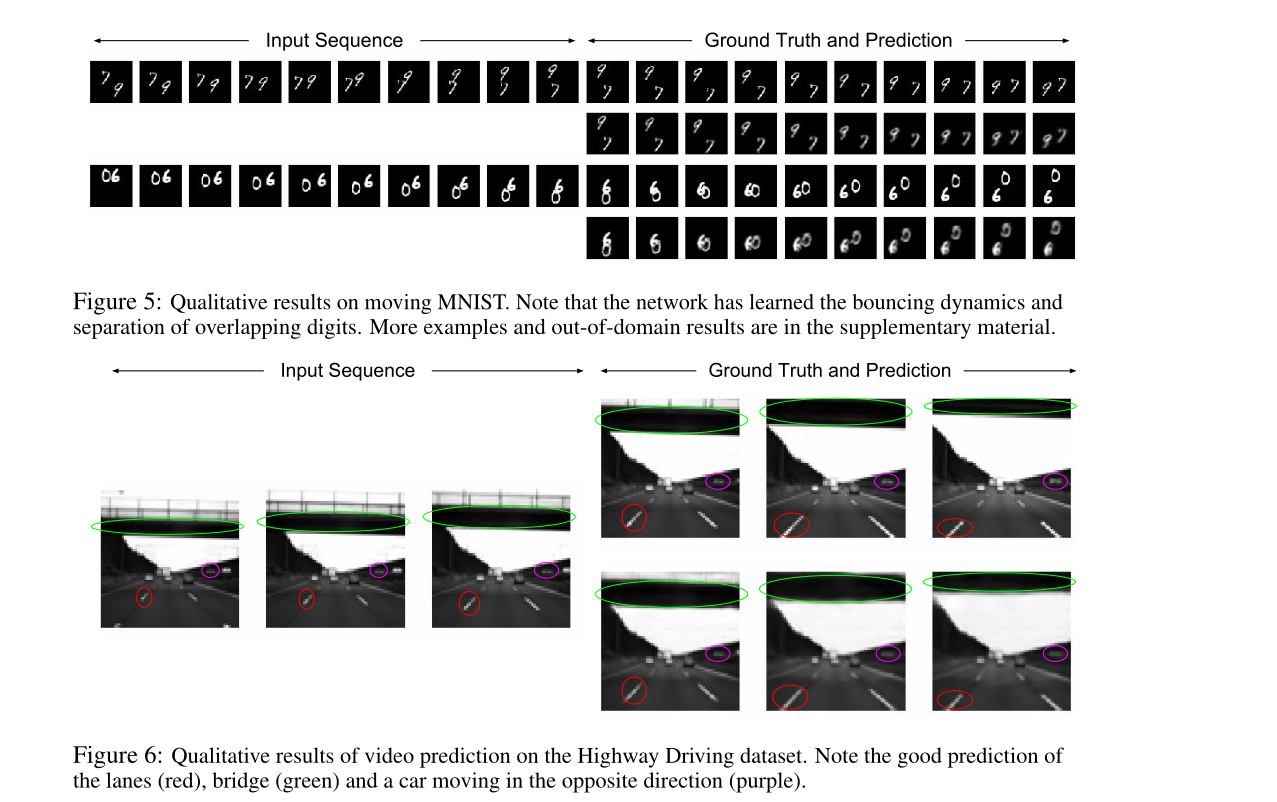卷积编码器-解码器有一个很大的接受域，这有助于模型学习弹跳行为，并生成正确的过滤器，以防数字弹跳墙壁。我们观察到预测随着时间的推移而恶化，即数字变得模糊。部分原因是由于模型误差:我们的模型不能在重叠后完美地分离数字，而且这些误差会随着时间累积。模糊的另一个原因来自数据集的人工制品:由于裁剪不完美，不确定数字何时会弹起并改变方向。这种行为不是完全确定的。这种不确定性加上像素上的损失函数，鼓励模型在数字到达边界时“对冲其赌注”，导致结果模糊。

总结

本文介绍了动态滤波网络，这是一类以特定于样本的方式将动态生成的滤波应用于图像的网络。作为未来的工作，我们计划探索动态过滤器网络在其他任务上的潜力，例如细粒度图像分类，过滤器可以学习适应对象姿态；或图像去模糊，过滤器可以调整适应图像结构。


展开全文• GPS动态滤波的理论方法及其应用 gps 地图匹配 卡尔曼滤波 等等
• 车载GPS导航系统动态滤波算法应用研究,硕士学位论文
• 如果将获取的权重叠加到卷积的kernel上就构成了动态滤波。方便对比理解所以将二者放到一起。 基于通道的注意力机制 基于通道的注意力机制比较经典的如SE-Net上用到的通道加权方式。 1、对特征实现全局平均池化得到一...
注意力机制
注意力机制主要分为两块，一块是基于通道的注意力机制，另一种是基于空间的空间注意力机制。结合二者的CBAM等。最近又有人提出Pixel Attention。这种方案我之后来讲。注意力机制的实际原理是通过特征图来计算特征图在通道和空间上的重要性。如果将获取的权重叠加到卷积的kernel上就构成了动态滤波。方便对比理解所以将二者放到一起。
基于通道的注意力机制
基于通道的注意力机制比较经典的如SE-Net上用到的通道加权方式。
1、对特征实现全局平均池化得到一维权重向量。
2、一维权重向量通过全连接和激活层等学习最终的权重向量。
3、将学习的权重向量对原来的特征实现加权。图1、通道加权的过程
示意代码如下：
import torch
import torch.nn as nn
class ChannelAttention(nn.Module):
def __init__(self, in_planes, ratio=16):
super(ChannelAttention, self).__init__()

self.fc1   = nn.Conv2d(in_planes, in_planes // 16, 1, bias=False)
self.relu1 = nn.ReLU()
self.fc2   = nn.Conv2d(in_planes // 16, in_planes, 1, bias=False)

self.sigmoid = nn.Sigmoid()

def forward(self, x):
avg_out = self.fc2(self.relu1(self.fc1(self.avg_pool(x))))
max_out = self.fc2(self.relu1(self.fc1(self.max_pool(x))))
out = avg_out + max_out
return self.sigmoid(out)
if __name__=="__main__":
x = torch.randn((1,64,32,32))
CA = ChannelAttention(in_planes=64)
cw = CA(x)
result = cw*x

基于空间的注意力机制
空间注意力机制，强调的是特征在空间分布上比较重要的区域：
1、计算特征的均值map和最大值map。
2、级联均值map和最大值map，将合并的特征送入卷积层学习得到空间权重再经过激活函数得到权重图。
3、空间权重图和特征图逐元素相乘。图2、空间注意力机制实现流程。我们简单的看看代码：
import torch
import torch.nn as nn
class SpatialAttention(nn.Module):
def __init__(self, kernel_size=7):
super(SpatialAttention, self).__init__()

assert kernel_size in (3, 7), 'kernel size must be 3 or 7'
padding = 3 if kernel_size == 7 else 1

self.sigmoid = nn.Sigmoid()

def forward(self, x):
avg_out = torch.mean(x,1).unsqueeze(1)
max_out, _ = torch.max(x,1).unsqueeze(1)
x = torch.cat([avg_out, max_out], dim=1)
x = self.conv1(x)
return self.sigmoid(x)
if __name__=="__main__":
x = torch.randn((1,64,32,32))
SA = SpatialAttention(kernel_size=3)
sw = SA(x)
result = sw*x

结合空间和通道的注意机制研究人员提出了CBAM:
CBAM的整体框架如下图3所示：直接将通道和空间注意力机制级联构成的，网络并没有考虑维度间的信息交互，为了改善这个问题，人们提出了Pixel Attention(像素级注意力机制)triplet Attention等。
Triplet Attention见下图所示。Triplet Attention由3个平行的Branch组成，其中两个负责捕获通道C和空间H或W之间的跨维交互。最后一个Branch类似于CBAM，用于构建Spatial Attention。最终3个Branch的输出使用平均进行聚合。简单的代码如下所示：
import torch
import torch.nn as nn
#z-pool
class ChannelPool(nn.Module):
def forward(self, x):
class BasicConv(nn.Module):
def __init__(self, in_planes, out_planes, kernel_size, stride=1, padding=0, dilation=1, groups=1, relu=True, bn=True, bias=False):
super(BasicConv, self).__init__()
self.out_channels = out_planes
self.bn = nn.BatchNorm2d(out_planes,eps=1e-5, momentum=0.01, affine=True) if bn else None
self.relu = nn.ReLU() if relu else None

def forward(self, x):
x = self.conv(x)
if self.bn is not None:
x = self.bn(x)
if self.relu is not None:
x = self.relu(x)
return x

class ChannelPool(nn.Module):
def forward(self, x):

class SpatialGate(nn.Module):
def __init__(self):
super(SpatialGate, self).__init__()
kernel_size = 7
self.compress = ChannelPool()
self.spatial = BasicConv(2, 1, kernel_size, stride=1, padding=(kernel_size-1) // 2, relu=False)
def forward(self, x):
x_compress = self.compress(x)
x_out = self.spatial(x_compress)
scale = torch.sigmoid_(x_out)
return x * scale

class TripletAttention(nn.Module):
def __init__(self, gate_channels, reduction_ratio=16, pool_types=['avg', 'max'], no_spatial=False):
super(TripletAttention, self).__init__()
self.ChannelGateH = SpatialGate()
self.ChannelGateW = SpatialGate()
self.no_spatial=no_spatial
if not no_spatial:
self.SpatialGate = SpatialGate()
def forward(self, x):
x_perm1 = x.permute(0,2,1,3).contiguous()
x_out1 = self.ChannelGateH(x_perm1)
x_out11 = x_out1.permute(0,2,1,3).contiguous()
x_perm2 = x.permute(0,3,2,1).contiguous()
x_out2 = self.ChannelGateW(x_perm2)
x_out21 = x_out2.permute(0,3,2,1).contiguous()
if not self.no_spatial:
x_out = self.SpatialGate(x)
x_out = (1/3)*(x_out + x_out11 + x_out21)
else:
x_out = (1/2)*(x_out11 + x_out21)
return x_out


动态滤波：
关于动态滤波实际上也将SE-Net的注意力模块的权重作用于用于卷积的卷积核而非特征图。由于权重的信息来自于特征图，这样导致滤波核与输入是相关的。因此，称为动态滤波。在我的另外一篇博客里面有简单的介绍了。这里就不再赘述了。Dynamic Convolution展开全文• 摘要：为了提高动态定位精度，将卡尔曼(KF)算法应用到GPS非线性动态定位解算中，提出加权最小二乘一卡尔曼滤波(WLS-KF)算法。
• SVC通用资料\GSTSF动态滤波无功补偿装置使用说明书.doc
• 最近在网上买了一块6050模块，用店家的给的代码，他用的是6050自带的dmp来直接得到姿态的 可是，如果拿着模块在空中剧烈翻转的话，就会有二十多度的误差，如果用卡尔曼滤波的话，会不会有这么严重的误差呢？三轴加速度传感器 传感器 单片机
• gps，卡尔曼动态滤波，gps滤波，gps动态滤波，gps静态滤波，
• 陈拓 2020/12/08-2020/12/19 1. 概述 在正弦波上叠加高斯白噪声，再用卡尔曼滤波滤除噪声。 相关文档： 《Echarts动态生成sin曲线》...《echarts动态滑动平均滤波》https://blog.csdn.ne
陈拓 2020/12/08-2020/12/19
1. 概述
在正弦波上叠加高斯白噪声，再用卡尔曼滤波滤除噪声。
相关文档：
《Echarts动态生成sin曲线》https://blog.csdn.net/chentuo2000/article/details/110622403
《Echarts动态产生高斯白噪声》https://blog.csdn.net/chentuo2000/article/details/110795146
《echarts动态滑动平均滤波》https://blog.csdn.net/chentuo2000/article/details/111088311
2. HTML页面和js程序
<!DOCTYPE html>
<html style="height: 100%">
<meta charset="utf-8">
<body style="height: 100%; margin: 0">
<div id="container1" style="position:absolute; left:10px; top:0px; height:320px; width:400px;"></div>
<div id="container3" style="position:absolute; left:400px; top:0px; height:320px; width:400px;"></div>
<div id="container5" style="position:absolute; left:800px; top:0px; height:320px; width:400px;"></div>
<div id="container6" style="position:absolute; left:800px; top:280px; height:320px; width:400px;"></div>
<script type="text/javascript" src="https://cdn.jsdelivr.net/npm/echarts@5/dist/echarts.min.js"></script>
<script type="text/javascript">
var dom1 = document.getElementById("container1");
var myChart1 = echarts.init(dom1);
var dom3 = document.getElementById("container3");
var myChart3 = echarts.init(dom3);
var dom5 = document.getElementById("container5");
var myChart5 = echarts.init(dom5);
var dom6 = document.getElementById("container6");
var myChart6 = echarts.init(dom6);
var app = {};
var option;

var N_POINT = 500; // 曲线的x轴长度
var angle = 0;
var x_axis1 = [];
var x_data1 = 0;
var y_axis1 = [];
var y_data1 = 0;
var x_axis3 = [];
var x_data3 = 0;
var y_axis3 = [];
var y_data3 = 0;
var x_axis5 = [];
var x_data5 = 0;
var y_axis5 = [];
var y_data5 = 0;
var x_axis6 = [];
var x_data6 = 0;
var y_axis6 = [];
var y_data6 = 0;

// 产生三角函数
function sin_data(x) {
x = x/10;
return Math.sin(x*Math.PI);
}

// 产生服从 U(0,1) 的均匀分布随机数random1和random2
function random1(x) {
return Math.random();
}
function random2(x) {
return Math.random();
}

// 用Box Muller方法生成正态分布随机数
function b_m(x, y) {
var means= 0; // 平均值，决定对称中线
var sigma= 1; // 标准方差，决定曲线的胖瘦
z = Math.sqrt(-2 * Math.log(x)) * Math.cos(2 * Math.PI * y);
return means + z * sigma;
}

/* 卡尔曼滤波
R值固定，Q值越大，代表越信任测量值，Q值无穷大，代表只用测量值。
Q值越小，代表越信任模型预测值，Q值为0，代表只用模型预测值。
Q增大，动态响应变快，收敛稳定性变坏
R增大，动态响应变慢，收敛稳定性变好
*/
var LastP = 0.02;//上次估算协方差初始化值为0.02
var Now_P = 0;//当前估算协方差初始化值为0
var out = 0;//卡尔曼滤波器输出初始化值为0
var Kg = 0;//卡尔曼增益初始化值为0
var Q = 0.0005;//过程噪声协方差初始化值为0.0005
var R = 0.6;//观测噪声协方差初始化值为0.543
function KalmanFilter(inData)
{
//预测协方差方程：k时刻系统估算协方差 = k-1时刻的系统协方差 + 过程噪声协方差
Now_P = LastP + Q;
//卡尔曼增益方程：卡尔曼增益 = k时刻系统估算协方差 / （k时刻系统估算协方差 + 观测噪声协方差）
Kg = Now_P/(Now_P + R);
//更新最优值方程：k时刻状态变量的最优值 = 状态变量的预测值 + 卡尔曼增益 * （测量值 - 状态变量的预测值）
out = out + Kg * (inData - out); //因为这一次的预测值就是上一次的输出值
//更新协方差方程: 本次的系统协方差付给 LastP 威下一次运算准备。
LastP = (1-Kg) * Now_P;
return out;
}

// 生成x轴和y轴数据
x_data1++;
x_axis1.push(x_data1);
x_data3++;
x_axis3.push(x_data3);
x_data5++;
x_axis5.push(x_data5);
x_data6++;
x_axis6.push(x_data6);

y_data1 = 10*sin_data(angle); // 生成sin曲线
y_axis1.push(y_data1);
angle+=0.1;

var x = random1(); // 生成均匀分布的伪随机数
var y = random2(); // 生成均匀分布的伪随机数
y_data3 = b_m(x, y); // 生成正态分布的随机数
y_axis3.push(y_data3);

y_data5 = y_data1 + y_data3; // 生成混合信号
y_axis5.push(y_data5);

y_data6 = KalmanFilter(y_data5)
y_axis6.push(y_data6);

if (shift) {
x_axis1.shift();
y_axis1.shift();
x_axis3.shift();
y_axis3.shift();
x_axis5.shift();
y_axis5.shift();
x_axis6.shift();
y_axis6.shift();
}
}
// 生成N_POINT个数据，初始化x_axis和y_axis数组，数组的长度在这里被设置成N_POINT
for (var i = 0; i < N_POINT; i++) {
}

option1 = {
title : {
show:true,
text: 'sin曲线',
x:'center'
},
xAxis: {
type: 'category',
data: x_axis1
},
yAxis: {
type: 'value'
},
series: [{
animation: false,
showSymbol: false,
clip: true,
data: y_axis1,
type: 'line'
}]
};

option3 = {
title : {
show:true,
text: '高斯白噪声',
x:'center'
},
xAxis: {
type: 'category',
data: x_axis3
},
yAxis: {
type: 'value'
},
series: [{
animation: false,
showSymbol: false,
clip: true,
data: y_axis3,
type: 'line'
}]
};

option5 = {
title : {
show:true,
text: '混合信号',
x:'center'
},
xAxis: {
type: 'category',
data: x_axis5
},
yAxis: {
type: 'value'
},
series: [{
animation: false,
showSymbol: false,
clip: true,
data: y_axis5,
type: 'line'
}]
};
option6 = {
title : {
show:true,
text: '卡尔曼滤波后的结果',
x:'center'
},
xAxis: {
type: 'category',
data: x_axis6
},
yAxis: {
type: 'value'
},
series: [
{
animation: false,
showSymbol: false,
type: 'line',
data: y_axis6,
smooth: true,
itemStyle: {
normal: {
lineStyle: {
color: '#c23531' //改变折线颜色
}
}
}
}
]
};

setInterval(function () {
myChart1.setOption(option1);
myChart3.setOption(option3);
myChart5.setOption(option5);
myChart6.setOption(option6);
}, 500); // 500ms刷新一次
</script>
</body>
</html>

3. 在浏览器中的效果图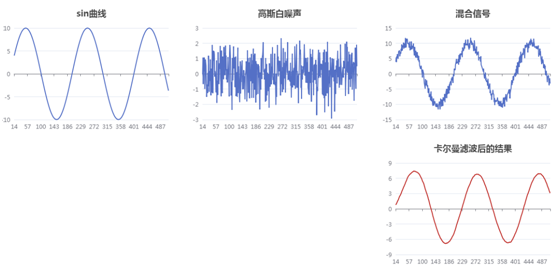可以调整Q和R值来观察不同的滤波效果。


展开全文echarts javascript
• 该文档基于动态线滤波较详细的描述了血液流动的可视化探索
• Idea opencv 中 有个实现 高斯滤波 的接口，...在做项目的过程中，我发现如果根据 像素点 相对整张图片 的位置 设计 不同的 滤波核大小（即参数 ksize），就可以灵活地对整张图片实现 动态 高斯滤波 了。 具体滤波
Idea

opencv 中 有个实现 高斯滤波 的接口，如下：

cv2.GaussianBlur(src, ksize, sigmaX, dst=None, sigmaY=None, borderType=None)


在做项目的过程中，我发现如果根据 像素点 相对整张图片 的位置 设计 不同的 滤波核大小（即参数 ksize），就可以灵活地对整张图片实现 动态 高斯滤波 了。

具体滤波核大小计算公式如下：

size = int(k1*x + k2*y + b) * 2 + 1


Note : 注意保证 输出结果 为 整型奇数，因为 参数 ksize 只 接受 整型奇数输入。

由于我的图片大小是 600×424×3 ，所以我的 k1，k2，b 取值如下：

k1,k2,b=⎧⎩⎨⎪⎪0,0.012,00.009,0,00.0053,0.0053,0垂直高斯;水平高斯;对角高斯.<!--//--><![CDATA[//><!--
k1, k2, b=\left\{\begin{array}{ll}0, 0.012, 0 &\mbox{垂直高斯;}\\0.009, 0, 0 &\mbox{水平高斯;}\\0.0053, 0.0053, 0 &\mbox{对角高斯.}\end{array}\right.
//--><!]]>Demo

原图像：水平动态高斯滤波：垂直动态高斯滤波：Code

以下代码中，关于　“对角动态高斯滤波（diagonal gaussian）”　的代码段有问题，所以被我注释掉了。
因为不能对单元素单独进行高斯滤波，所以会报错。具体该怎么解决，我也一时没想到。

# coding=utf-8

import numpy as np
import cv2

def kernel_size(x, y, type):
if type == "vertical":
k1, k2, b = 0, 0.012, 0
elif type == "horizontal":
k1, k2, b = 0.009, 0, 0
elif type == "diagonal":
k1, k2, b = 0.0053, 0.0053, 0
else:
k1, k2, b = 0, 0, 11
size = int(k1*x + k2*y + b) * 2 + 1
return size

def range_limit(img):
img *= (img>0)
img = img * (img<=255) + 255 * (img>255)
img = img.astype(np.uint8)
return img

def dynamic_gaussian(img):
h, w, c = np.shape(img)
sigma = 2
# gs_v: vertical gaussian, 垂直高斯
# gs_h: horizontal gaussian, 水平高斯
# gs_d: diagonal gaussian, 对角高斯
gs_v, gs_h, gs_d = img.copy(), img.copy(), img.copy()
for i in range(h):
k_size = kernel_size(w, i, "vertical")
gs_v[i, :, :] = cv2.GaussianBlur(gs_v[i, :, :], (k_size, k_size), sigma)
print(i, k_size)
for j in range(w):
k_size = kernel_size(j, h, "horizontal")
gs_h[:, j, :] = cv2.GaussianBlur(gs_h[:, j, :], (k_size, k_size), sigma)
print(j, k_size)
# # 报错：
# for i in range(h):
#     for j in range(w):
#         k_size = kernel_size(i, j, "diagonal")
#         gs_d[i, j, :] = cv2.GaussianBlur(gs_d[i, j, :], (k_size, k_size), sigma)
#         print(i, j, k_size)
return gs_v, gs_h, gs_d

def main():
img_path = "./girl.jpg"
gs_v, gs_h, gs_d = dynamic_gaussian(img)
cv2.imwrite("./gs_v.jpg", gs_v)
cv2.imwrite("./gs_h.jpg", gs_h)
cv2.imwrite("./gs_d.jpg", gs_d)

if __name__ == "__main__":
main()
展开全文• 详细介绍GPS动态卡尔曼滤波算法研究，介绍了卡尔曼滤波原理等
• 相关文档：晨之清风：Echarts动态生成sin曲线​zhuanlan.zhihu.com晨之清风：Echarts动态产生高斯白噪声​zhuanlan.zhihu.com晨之清风：echarts动态滑动平均滤波​zhuanlan.zhihu.com2. HTML页面和js程序<...
• 旨在通过简单的匀速直线运动场景，理解Kalman滤波过程，运行结果可参考本博主的《卡尔曼滤波（Kalman Filter）》
• 1、HMM：隐马尔可夫过程 2、线性动态系统：卡尔曼滤波 3、非线性动态系统：粒子滤波 对于HMM（隐马尔可夫模型），常与EM算法联系使用。模型最终转化为求取参数,其中代表初始概率 ， A代表状态转移矩阵，B代表...
• 一种改进的GPS动态定位滤波方法,针对GPS接收机能够测量卫星信号的多普勒频率的特性，亦即能够测量l_维速度的特性，引人了一种 改进的GPS定位系统滤波算法。首先，根据机动载体的“当前”统计模型建立了系统状态方程...
• 采用FC+SVG并联装置抑制无功功率及高次谐波的方法,对煤矿供电系统采用动态补偿滤波。通过该技术的应用,可以快速地跟随矿井负荷无功电流的变化而变化,自动补偿电网系统所需无功功率,对电网无功功率实现动态无级补偿。...
• 在做课程设计的时候遇到了运用卡尔曼滤波进行移动目标跟踪问题，对此参考了很多资源，完成了题目要求，如果有做卡尔曼滤波的可以放心下载，有详尽注释。
• 《Echarts动态生成sin曲线》https://blog.csdn.net/chentuo2000/article/details/110622403 《Echarts动态产生高斯白噪声》https://blog.csdn.net/chentuo2000/article/details/110795146 2. HTML页面和js程序 &...滑动平均 javascript
•研究论文
• 该文档详细分析了，动态定位的卡尔曼滤波的应用和研究
• 功能：将串口数据接收后...2、解析后的数据，动态滤波 3、滤波前后的数据都显示出来，便于对比。 文件列表 fft:将保存的数据用此函进行频谱分析。 MyFilter：修改滤波系数 serialApi:串口接收函烽 testFilt
• 数字信号滤波的方式，对原始信号有很多种滤波方式，包括小波滤波方式，FIR滤波，IIR滤波，LMS自适应滤波，代码是用C语言写的。
• 为了实现工业相机对动态目标的准确、实时跟踪，提出了基于卡尔曼滤波的算法。通过创建背景模型来估计出当前背景，进而得到前景区域，并对前景区域进行相关处理，最后通过计算补集得到更新后的背景。此方法能根据不同......